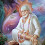### Calculation of power generation cost of Thermal power plants & Co-generation plants

Power generation cost: It is the amount of rupees spent on generation of 1 unit of power

Power export cost: It is the amount of rupees spent on export of 1 unit of power

Generally power generation actual cost is the sum of Fuel cost, man power cost, operation & maintenance costs administration cost, river water cost, plant gardening & vehicles cost  etc

Power generation & Export cost in Thermal power plants:

Part A:Fixed data
• Total power generation in KWH = A1
• Total power export KWH = A2
• Total fuel consumed = A3
• Rate of per ton of fuel in = A4
• Raw water consumption per day in MT or M3 = A5
• Rate of per ton or   of river water in  = A6
• Rate of per unit power for lifting water from source to Reservoir Rs= A7

Part B:
• Cost of fuel in Rs B1= A3 X A4
• Cost of raw water in Rs B2 = A5 X A6
• Cost of chemicals consumed for water treatment = B3
• Cost of fuel feeding in =B4
• Cost of raw water lifting charges from river to reservoir= B5
Total operation cost C = B1 + B2 +B3 + B4 + B5

Part C:
• Cost of spares,consumables consumed  = C1
• Cost of Lubricants = C2
• Cost of store inventory= C3
• Tools tackles testing cost = C4
• Measuring instruments calibration cost = C5
Total maintenance cost C = C1 +C2 + C3 + C4 + C5

Part D :
• Cost of  O &M man power salary = D1

• Cost of site expenditures =  D2

• Cost of gardening labours salary =D3

• Cost of Security guards salary = D4

• Cost of transportation vehicles =D5
Total administration cost = D = D1 + D2 + D3 + D4 + D5
• Total Cost of production, E = B + C + D
•
• Power generation cost per unit =A1/E....Rupees/KWh or Dollars/Kwh

• Power export cost per unit = A2/E.....Rupees/KWh or Dollars/Kwh

Note:

1-In calculation power generation cost, power consumed for plant auxiliary running should not be considered

2-In co-generation plants:
• Cost of power given to process plants should be considered
• Cost of process steam given should be considered for power generation cost calculation

1.How to calculate a power generation by generator in Hydro power plant?

1.Hyd.power geration = Flow in M3/sec X Net head (m) X 9.81 m/sec2 X Water density (kg/m3) / (1000 X Turbine eff. X Generator eff).....

.KW

2.How to calculate coal cost for Thermal power plant?

Hi all,